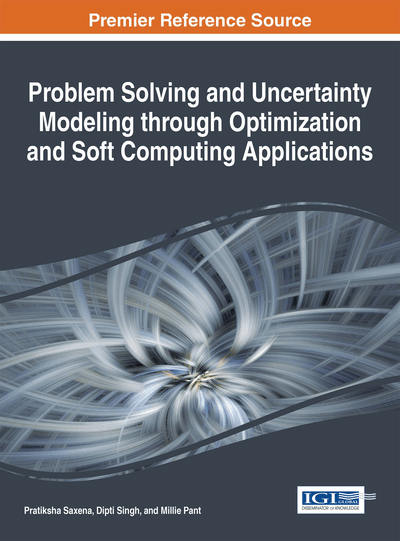# A Novel Mutation Strategy for Differential Evolution

Hira Zaheer (Indian Institute of Technology Roorkee, India), Millie Pant (Indian Institute of Technology Roorkee, India), Sushil Kumar (Amity University, India) and Oleg Monakhov (Institute of Computational Mathematics and Mathematical Geophysics, Russia)
DOI: 10.4018/978-1-4666-9885-7.ch002

## Abstract

Differential Evolution (DE) has attained the reputation of a powerful optimization technique that can be used for solving a wide range of problems. In DE, mutation is the most important operator as it helps in generating a new solution vector. In this paper we propose an additional mutation strategy for DE. The suggested strategy is named DE/rand-best/2. It makes use of an additional parameter called guiding force parameter K, which takes a value between (0,1) besides using the scaling factor F, which has a fixed value. De/rand-best/2 makes use of two difference vectors, where the difference is taken from the best solution vector. One vector difference will be produced with a randomly generated mutation factor K (0,1). It will add a different vector to the old one and search space will increase with a random factor. Result shows that this strategy performs well in comparison to other mutation strategies of DE.
Chapter Preview
Top

## 1. Introduction

Many real world applications Storn and Price (1997), Price et al. (2006), Joshi and Sanderson (1999), Zhang et al. (2008), Zhang et al. (2010), shows that Differential Evolution (DE) is an efficient algorithm for dealing with a variety of problems. DE was first introduced by Storn and Price in 1995, with the strategy DE/rand/1/bin which is still widely used among the researchers. Gämperle et al. (2002) and Zhang et al. (2007) shows that the performance of DE mostly depends on mutation factor and crossover probability. So, a change in these parameters affects the performance of DE in all aspects. Later on, different DE strategies were proposed by Storn (1996), Price (1999) and it was observed by Storn and Price (1997) and Gämperle et al. (2002) that the strategies in which there are two difference vectors such as DE/rand/2/bin and DE/best/2/bin are better than the strategies having difference vector, as diversity increases by adding more trial vectors as stated by Lampinen and Zelinka (2000). DE/best/1/bin and DE/rand-to-best/1/bin converges fast on easy optimization problems, but become reckless for highly multi-modal problems. DE/target-to-best/1/bin variant was proposed with the concept of neighborhood of each population so as to balance exploration and exploitation Das et al. (2009). DE/current-to-rand/1/bin can solve rotated problems more easily as compared to other strategies, Iorio and Li (2005).

A lot of research has been done to further enhance the performance of DE. Researchers have experimented with the setting of parameters Price et al. (2006), Gämperle et al. (2002), Gämperle (2006) and have also suggested adaptive and self-adaptive DE algorithms Qin and Suganthan (2005) and Huang et al. (2006). Some recently suggested DE variants and their applications in various engineering fields are given in Box 1.

## Complete Chapter List

Search this Book:
Reset